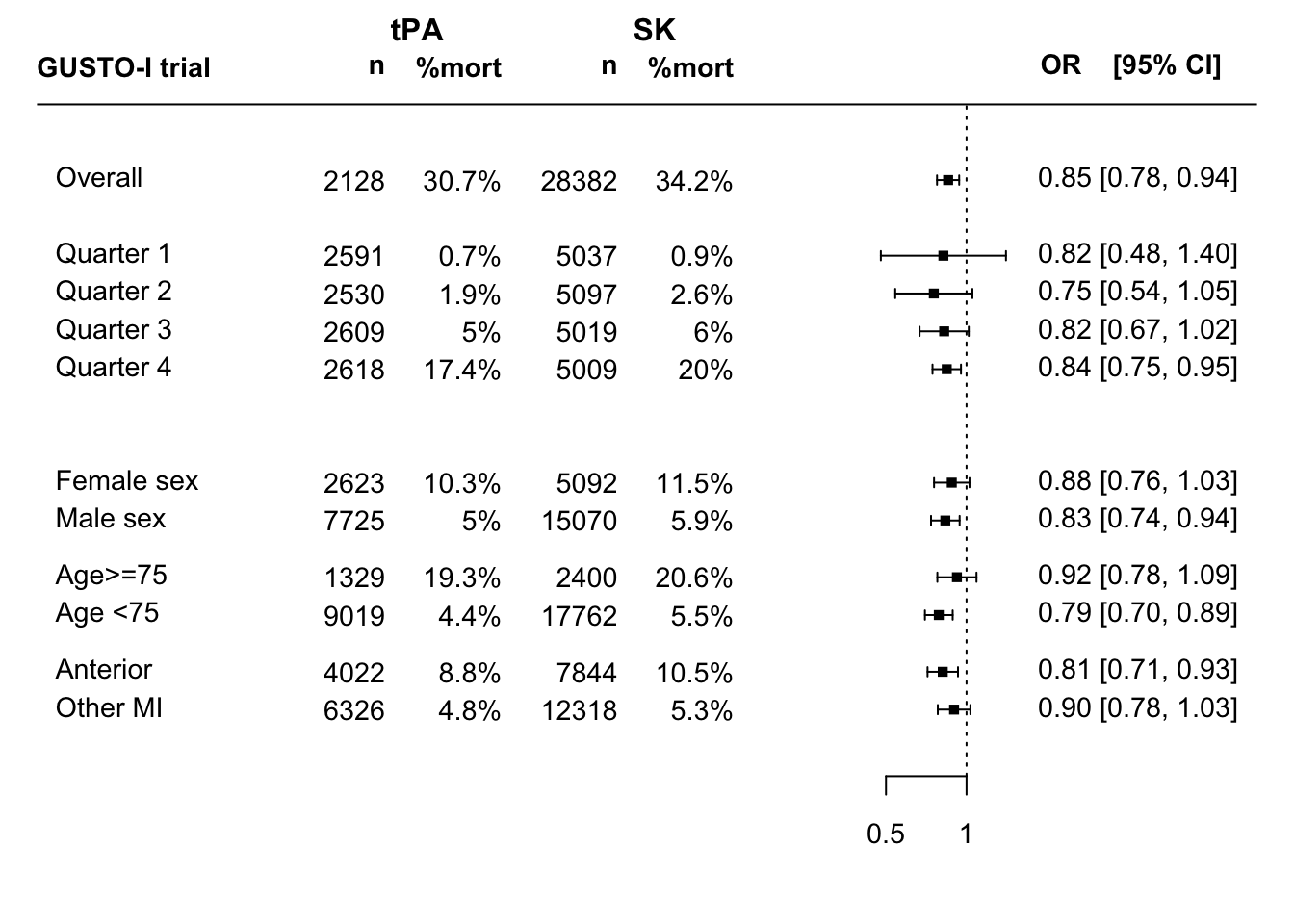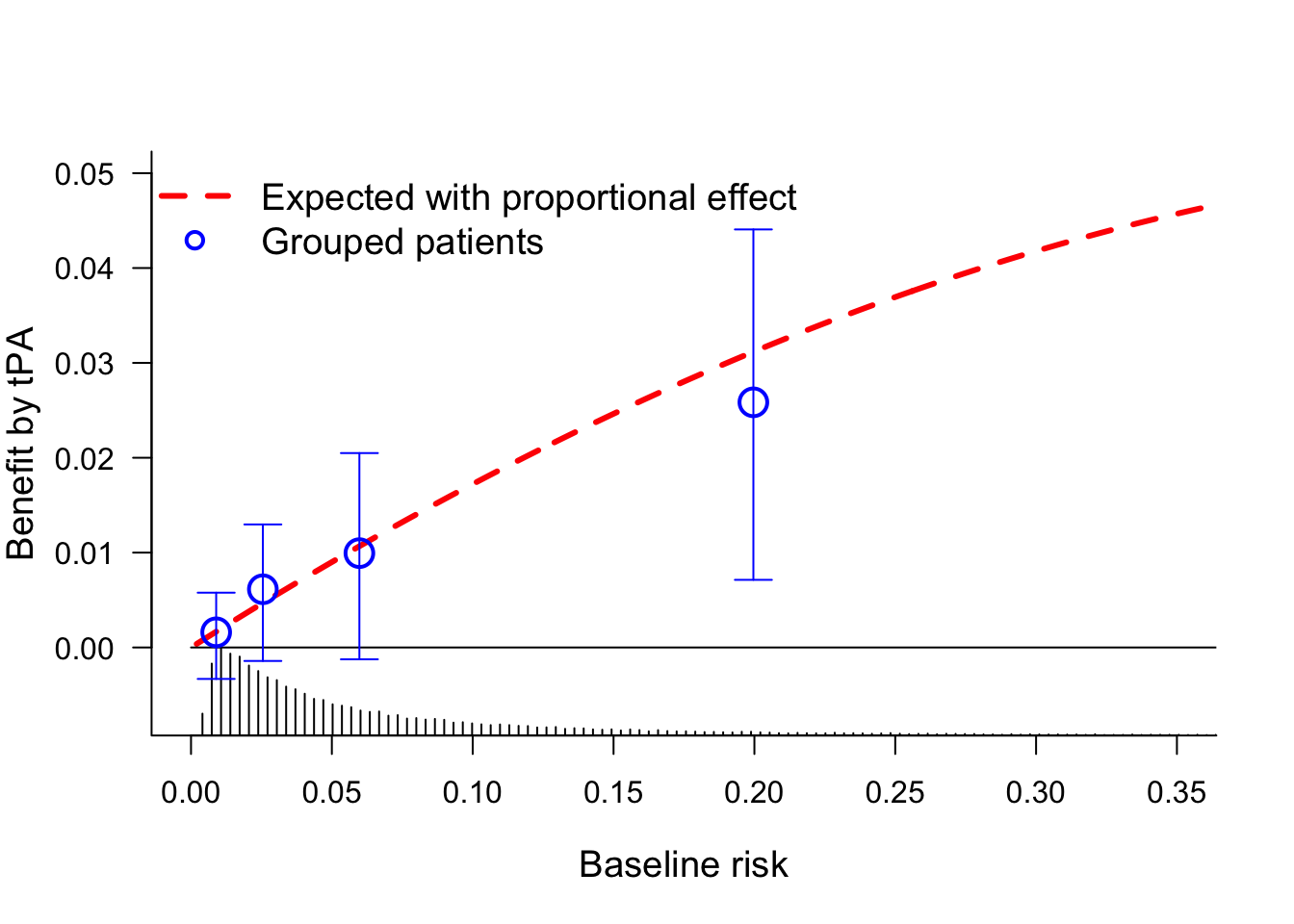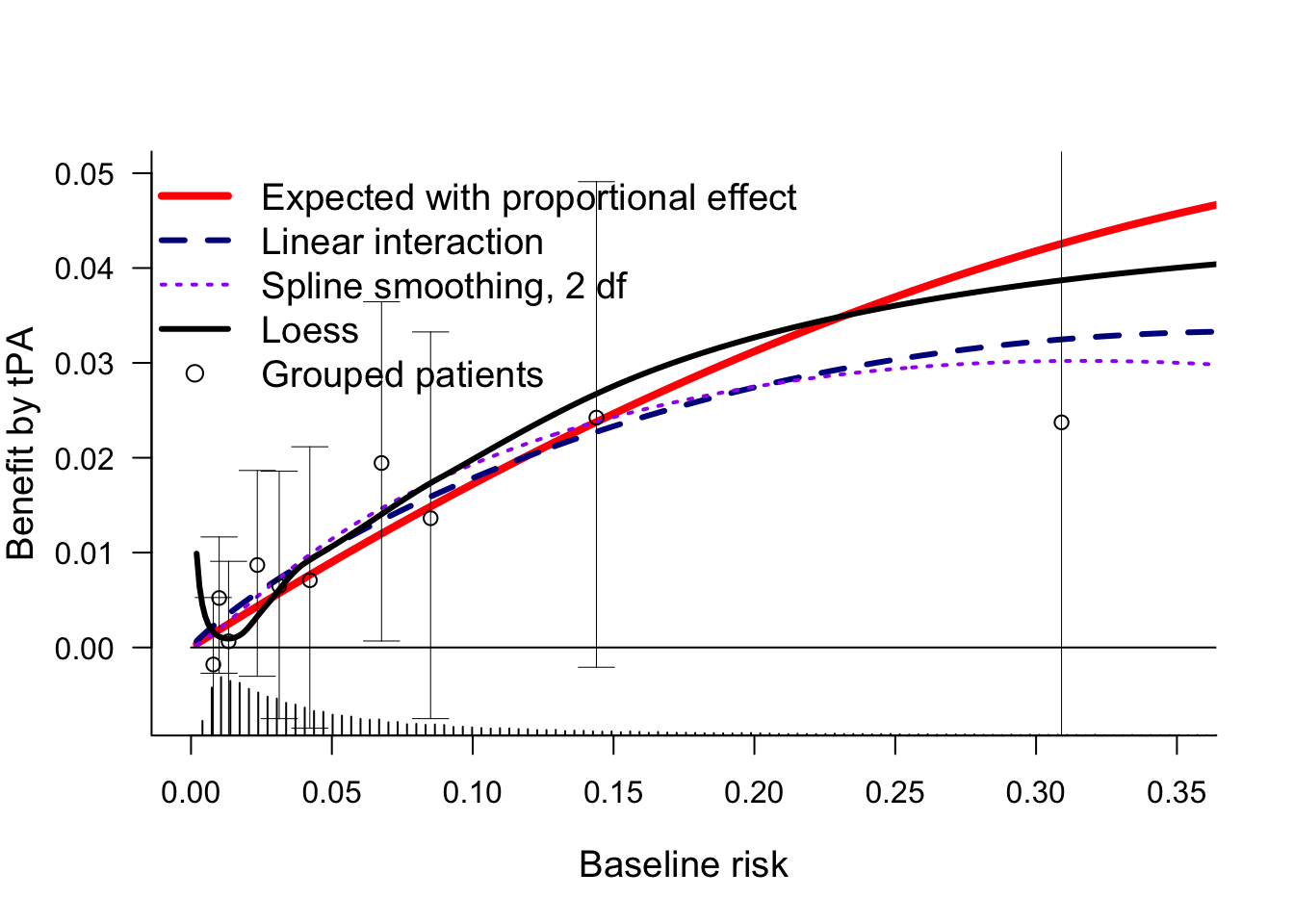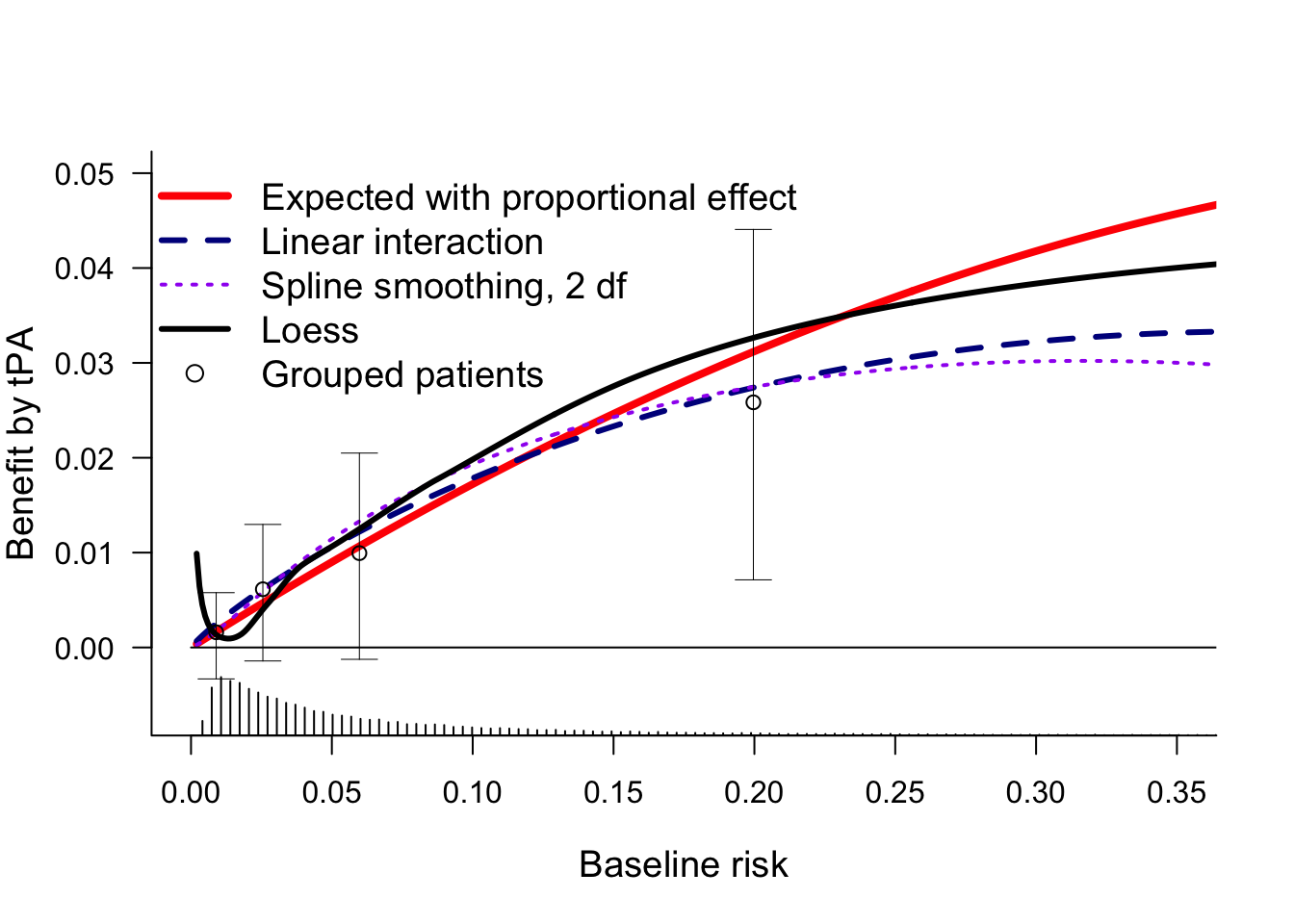# Implementation of the PATH Statement

The recent PATH (Predictive Approaches to Treatment effect Heterogeneity) Statement outlines principles, criteria, and key considerations for applying predictive approaches to clinical trials to provide patient-centered evidence in support of decision making. Here challenges in implementing the PATH Statement are addressed with the GUSTO-I trial as a case study.
Author
Affiliation

Department of Biomedical Data Sciences
Leiden University Medical Center NL
`@ESteyerberg`

Published

November 24, 2020

Evidence is derived from groups while most medical decisions are made for individual patients. — Kent et al, PATH statement

Heterogeneity of treatment effect (HTE) refers to the nonrandom variation in the magnitude of the absolute treatment effect (treatment benefit) across individual patients. The recent PATH (Predictive Approaches to Treatment effect Heterogeneity) Statement outlines principles, criteria, and key considerations for applying predictive approaches to clinical trials to provide patient-centered evidence in support of decision making. The focus of PATH is on modeling of HTE across individual patients.

The PATH statement lists a number of principles and guidelines. A first principle is to establish overall treatment effect. In another blog, I summarized the arguments in favor of covariate adjustment as the primary analysis of a RCT. Illustration was in the GUSTO-I trial. Here I continue that illustration, also following the blog by Frank Harrell on examining HTE.

## Illustration in the GUSTO-I trial

Let’s analyze the data from 30,510 patients with an acute myocardial infarction as included in the GUSTO-I trial. In GUSTO-I, 10,348 patients were randomized to receive tissue plasminogen activator (tPA), while 20,162 were randomzied to Streptokinase (SK) and had 30-day mortality status known.

Code
``````load(url('http://hbiostat.org/data/gusto.rda'))
# keep only SK and tPA arms; and selected set of covariates
gusto <- upData(gusto[gusto\$tx=="SK" | gusto\$tx=="tPA",],
keep=Cs(day30, tx, age, Killip, sysbp, pulse, pmi, miloc, sex),
print=FALSE)
html(describe(gusto), scroll=TRUE)``````
gusto Descriptives
gusto

9 Variables   30510 Observations

day30
 n missing distinct Info Sum Mean Gmd 30510 0 2 0.195 2128 0.06975 0.1298

sex: Sex

 n missing distinct 30510 0 2
`````` Value        male female
Frequency   22795   7715
Proportion  0.747  0.253
``````

Killip: Killip Classn missing distinct 30510 0 4
`````` Value          I    II   III    IV
Frequency  26007  3857   417   229
Proportion 0.852 0.126 0.014 0.008
``````

agen missing distinct Info Mean Gmd 0.05 0.1 0.25 0.5 0.75 0.9 0.95 30510 0 5342 1 60.91 13.58 40.92 44.73 52.11 61.58 69.84 76.19 79.42

lowest : 19.0 20.8 21.0 21.0 21.4 , highest: 91.9 92.3 96.5 108.0 110.0

pulse: Heart Rate beats/minn missing distinct Info Mean Gmd 0.05 0.1 0.25 0.5 0.75 0.9 0.95 30510 0 157 0.999 75.38 19.5 50 55 62 73 86 98 107

lowest : 0 1 6 9 20 , highest: 191 200 205 210 220

sysbp: Systolic Blood Pressure mmHgn missing distinct Info Mean Gmd 0.05 0.1 0.25 0.5 0.75 0.9 0.95 30510 0 196 0.999 129 26.58 92 100 112 129.5 144 160 170

lowest : 0 36 40 43 46 , highest: 266 274 275 276 280

miloc: MI Locationn missing distinct 30510 0 3
`````` Value      Inferior    Other Anterior
Frequency     17582     1062    11866
Proportion    0.576    0.035    0.389
``````

pmi: Previous MI

 n missing distinct 30510 0 2
`````` Value         no   yes
Frequency  25452  5058
Proportion 0.834 0.166
``````

tx: Tx in 3 groups

 n missing distinct 30510 0 2
``` Value         SK   tPA
Frequency  20162 10348
Proportion 0.661 0.339
```

### Overall treatment effect

The primary outcome was 30-day mortality. Among the tPA group, the 30-day mortality was 653/10,348 = 6.3% vs 1475/20,162 = 7.3% in the SK group. This an absolute difference of 1.0%, or an odds ratio of 0.85 [0.78-0.94].

Code
``````# simple cross-table
table1(~ as.factor(day30) | tx, data=gusto, digits=2)  ``````
SK
(N=20162)
tPA
(N=10348)
Overall
(N=30510)
as.factor(day30)
0 18687 (92.7%) 9695 (93.7%) 28382 (93.0%)
1 1475 (7.3%) 653 (6.3%) 2128 (7.0%)
Code
``````# 'tpa' as 0/1 variable for tPA vs SK treatment
gusto\$tx <- droplevels(gusto\$tx) # Drop the SK + tPA category which has 0 obs
gusto\$tpa <- as.numeric(gusto\$tx) - 1 # 0/1 coding for tPA treatment
label(gusto\$tpa) <- "tPA"
levels(gusto\$tpa) <- c("SK", "tPA")
tab2 <- table(gusto\$day30, gusto\$tpa)
result <- OddsRatio(tab2, conf.level = 0.95)
names(result) <- c("Odds Ratio", "Lower CI", "Upper CI")

kable(as.data.frame(t(result))) %>% kable_styling(full_width=F, position = "left")``````
Odds Ratio Lower CI Upper CI
0.853 0.776 0.939
Code
``````# BinomDiffCI(x1 = events1, n1 = n1, x2 = events2, n2 = n2, ...)
CI      <- BinomDiffCI(x1 = tab2[2,1], n1 = sum(tab2[,1]), x2 = tab2[2,2], n2 = sum(tab2[,2]),
method = "scorecc")
colnames(CI) <- c("Absolute difference", "Lower CI", "Upper CI")

result <- round(CI, 3) # absolute difference with confidence interval
kable(as.data.frame(result)) %>% kable_styling(full_width=F, position = "left")``````
Absolute difference Lower CI Upper CI
0.01 0.004 0.016

The unadjusted odds ratio of 0.853 is a marginal estimate. As explained in the other blog, a lot can be said in favor of conditional estimates, where we adjust for prognostically important baseline characteristics. In line with Califf 1997 and Steyerberg 2000, we consider a prediction model with 6 baseline covariates, including age and Killip class (a measure for ventricular function). Pulse rate is modeled using a linear spline with a knot at 50 beats/minute.

Code
``````options(prType='html')
f <- lrm(day30 ~ tpa + age + Killip + pmin(sysbp, 120) + lsp(pulse, 50) + pmi + miloc, data=gusto, x=T, maxit=99)
print(f) # coef tPA: -0.2080``````
Logistic Regression Model
``` lrm(formula = day30 ~ tpa + age + Killip + pmin(sysbp, 120) +
lsp(pulse, 50) + pmi + miloc, data = gusto, x = T, maxit = 99)
```
Model Likelihood
Ratio Test
Discrimination
Indexes
Rank Discrim.
Indexes
Obs 30510 LR χ2 2991.95 R2 0.235 C 0.815
0 28382 d.f. 11 R211,30510 0.093 Dxy 0.631
1 2128 Pr(>χ2) <0.0001 R211,5938.7 0.395 γ 0.631
max |∂log L/∂β| 6×10-10 Brier 0.056 τa 0.082
β S.E. Wald Z Pr(>|Z|)
Intercept  -3.0203  0.7973 -3.79 0.0002
tpa  -0.2080  0.0529 -3.93 <0.0001
age   0.0769  0.0025 31.28 <0.0001
Killip=II   0.6137  0.0589 10.42 <0.0001
Killip=III   1.1610  0.1214 9.57 <0.0001
Killip=IV   1.9213  0.1618 11.87 <0.0001
sysbp  -0.0392  0.0019 -20.33 <0.0001
pulse  -0.0242  0.0159 -1.52 0.1282
pulse'   0.0433  0.0162 2.67 0.0075
pmi=yes   0.4472  0.0562 7.96 <0.0001
miloc=Other   0.2863  0.1347 2.13 0.0335
miloc=Anterior   0.5432  0.0511 10.62 <0.0001

So, we note that the adjusted regression coefficient for tPA was -0.208. The adjusted OR = 0.812.
Let’s check for statistical interaction with

1. Individual covariates
2. The linear predictor
Code
``````## check for interaction
g <- lrm(day30 ~  tx * (age + Killip + pmin(sysbp, 120) + lsp(pulse, 50) + pmi + miloc),
data=gusto, maxit=100)
print(anova(g)) # tx interactions: 10 df, p=0.5720; based on LR test``````
Wald Statistics for `day30`
χ2 d.f. P
tx (Factor+Higher Order Factors) 23.48 11 0.0151
All Interactions 8.53 10 0.5772
age (Factor+Higher Order Factors) 981.65 2 <0.0001
All Interactions 1.60 1 0.2065
Killip (Factor+Higher Order Factors) 281.44 6 <0.0001
All Interactions 1.37 3 0.7131
sysbp (Factor+Higher Order Factors) 412.30 2 <0.0001
All Interactions 0.11 1 0.7369
pulse (Factor+Higher Order Factors) 235.92 4 <0.0001
All Interactions 4.22 2 0.1214
Nonlinear (Factor+Higher Order Factors) 7.37 2 0.0251
pmi (Factor+Higher Order Factors) 64.23 2 <0.0001
All Interactions 0.05 1 0.8233
miloc (Factor+Higher Order Factors) 115.23 4 <0.0001
All Interactions 2.99 2 0.2239
tx × age (Factor+Higher Order Factors) 1.60 1 0.2065
tx × Killip (Factor+Higher Order Factors) 1.37 3 0.7131
tx × sysbp (Factor+Higher Order Factors) 0.11 1 0.7369
tx × pulse (Factor+Higher Order Factors) 4.22 2 0.1214
Nonlinear 0.08 1 0.7784
Nonlinear Interaction : f(A,B) vs. AB 0.08 1 0.7784
tx × pmi (Factor+Higher Order Factors) 0.05 1 0.8233
tx × miloc (Factor+Higher Order Factors) 2.99 2 0.2239
TOTAL NONLINEAR 7.37 2 0.0251
TOTAL INTERACTION 8.53 10 0.5772
TOTAL NONLINEAR + INTERACTION 15.57 11 0.1579
TOTAL 2343.20 21 <0.0001
Code
``````# 2. PATH statement: linear interaction with linear predictor; baseline risk, so tx=ref, SK
lp.no.tx <- f\$coefficients + 0 * f\$coefficients + f\$x[,-1] %*% f\$coefficients[-(1:2)]
gusto\$lp <- as.vector(lp.no.tx) # add lp to data frame
h <- lrm(day30 ~ tx * lp, data=gusto)
print(anova(h)) # tx interaction: 1 df, p=0.35; based on Wald statistics``````
Wald Statistics for `day30`
χ2 d.f. P
tx (Factor+Higher Order Factors) 16.24 2 0.0003
All Interactions 0.86 1 0.3526
lp (Factor+Higher Order Factors) 2338.37 2 <0.0001
All Interactions 0.86 1 0.3526
tx × lp (Factor+Higher Order Factors) 0.86 1 0.3526
TOTAL 2342.20 3 <0.0001

So:

1. The overall test for interaction with the individual covariates is far from statistically significant (p>0.5).
2. Similarly, the test for interaction with the linear predictor is far from statistically significant (p>0.3).

#### Conclusion: no interaction needed

We conclude that we may proceed by ignoring any interactions. We have no evidence against the assumption that the overall effect of treatment is applicable to all patients.
The patients vary widely in risk, as can easily be seen in the histogram below.

Code
``hist(plogis(lp.no.tx), xlim=c(0,.4), main="")``We note that many patients have baseline risks (tPA==0) below 5%. Obviously their maximum benefit is bounded by this risk estimate, even if tPA, hypothetically, would reduce the risk to Null.

## PATH principle: perform risk-based subgrouping

Fig 3 of the PATH Statement starts with:

Reporting RCT results stratified by a risk model is encouraged when overall trial results are positive to better understand the distribution of effects across the trial population.

How do we provide such reporting of RCT results?

We can provide estimates of relative effects and absolute benefit

1. By group (e.g. quarters defined by quartiles)
2. By baseline risk (continuous, as in the histogram above)

### 1a. Relative effects of treatment by risk-group

The checks for interaction were far from statistically significant in GUSTO-I. We can further illustrate the relative effects in a forest plot. Many reports from RCTs include forest plots that show relative effects by single variables, such as men vs women; young vs old age; disease subtype; etc.
The PATH statement encourages reporting by risk-based subgroup. How can such reporting be done?

Let’s do some data processing to make a better forest plot.

#### A PATH compatible forest plot

Let’s expand the standard forest plot for subgroup effects with risk-based subgroups.
Below subgroup effects are defined for 4 risk-based groups using `cut2(lp.no.tx, g=4)`,
and for 3 classical subgroups (by sex, age, type of infarction).

Code
``````## quantiles, suggest to use quarters
groups <- cut2(lp.no.tx, g=4)
group0 <- groups[gusto\$tpa==0]  # SK gropup
group1 <- groups[gusto\$tpa==1]  # tPA group

rate0 <- prop.table(table(group0, gusto\$day30[gusto\$tpa==0]),1 )[,2]
rate1 <- prop.table(table(group1, gusto\$day30[gusto\$tpa==1]),1 )[,2]
ratediff <- rate0-rate1 # benefit of tPA by group

# Make a data frame for the results
data.subgroups <- as.data.frame(matrix(nrow=(4+6+1), ncol=10))
colnames(data.subgroups) <- c("tevent", "tnoevent", "cevent", "cnoevent",
"name", "type", "tn", "pt", "cn", "pc")

data.subgroups[11,1:4] <- table(gusto\$tpa,gusto\$day30)[4:1] # overall results
# define event and non-event numbers
events1   <- table(group0, gusto\$day30[gusto\$tpa==0])[,2]
nevents1  <- table(group0, gusto\$day30[gusto\$tpa==0])[,1]
events2   <- table(group1, gusto\$day30[gusto\$tpa==1])[,2]
nevents2  <- table(group1, gusto\$day30[gusto\$tpa==1])[,1]
n1      <- events1 + nevents1
n2      <- events2 + nevents2

data.subgroups[10:7,1:4] <- cbind(events2,nevents2,events1,nevents1)``````

Data for classic subgroups are managed below:

Code
``````# Use `table`  to get the summary of cell numbers, by subgroup
# SEX
data.subgroups[5,1:4] <- table(1-gusto\$day30,1-gusto\$tpa, gusto\$sex)[1:4]
data.subgroups[6,1:4] <- table(1-gusto\$day30,1-gusto\$tpa, gusto\$sex)[5:8]
# AGE
data.subgroups[3,1:4] <- table(1-gusto\$day30,1-gusto\$tpa, gusto\$age>=75)[1:4]
data.subgroups[4,1:4] <- table(1-gusto\$day30,1-gusto\$tpa, gusto\$age>=75)[5:8]
# ANT
data.subgroups[1,1:4] <- table(1-gusto\$day30,1-gusto\$tpa, gusto\$miloc=="Anterior")[1:4]
data.subgroups[2,1:4] <- table(1-gusto\$day30,1-gusto\$tpa, gusto\$miloc=="Anterior")[5:8]

# Names
data.subgroups[11,5]   <- "Overall"
data.subgroups[10:7,5] <- paste("Quarter",1:4, sep=" ")
data.subgroups[5:6,5]  <- c("Male sex","Female sex")
data.subgroups[3:4,5]  <- c("Age <75","Age>=75")
data.subgroups[1:2,5]  <- c("Other MI","Anterior")

# Type of subgroup
data.subgroups[11,6]   <- ""
data.subgroups[10:7,6] <- c(rep("Risk-based subgroups", length(ratediff)))
data.subgroups[1:6,6] <- c(rep("Location",2), rep("Age",2), rep("Sex",2))

data.subgroups[,7] <- data.subgroups[,1] + data.subgroups[,2]
data.subgroups[,8] <- paste(round(100*data.subgroups[,1] / data.subgroups[,7] , 1),"%", sep="")
data.subgroups[,9] <- data.subgroups[,3] + data.subgroups[,4]
data.subgroups[,10] <- paste(round(100*data.subgroups[,3] / data.subgroups[,9] , 1),"%", sep="")

# Show the data
kable(as.data.frame((data.subgroups))) %>% kable_styling(full_width=F, position = "left")``````
tevent tnoevent cevent cnoevent name type tn pt cn pc
301 6025 649 11669 Other MI Location 6326 4.8% 12318 5.3%
352 3670 826 7018 Anterior Location 4022 8.8% 7844 10.5%
397 8622 981 16781 Age <75 Age 9019 4.4% 17762 5.5%
256 1073 494 1906 Age>=75 Age 1329 19.3% 2400 20.6%
383 7342 888 14182 Male sex Sex 7725 5% 15070 5.9%
270 2353 587 4505 Female sex Sex 2623 10.3% 5092 11.5%
455 2163 1000 4009 Quarter 4 Risk-based subgroups 2618 17.4% 5009 20%
130 2479 300 4719 Quarter 3 Risk-based subgroups 2609 5% 5019 6%
49 2481 130 4967 Quarter 2 Risk-based subgroups 2530 1.9% 5097 2.6%
19 2572 45 4992 Quarter 1 Risk-based subgroups 2591 0.7% 5037 0.9%
653 1475 9695 18687 Overall 2128 30.7% 28382 34.2%

In this table tevent means #events among treated; cevent means #events among non-treated; etc

Results can be plotted with `metafor` functions:

Code
``````par(mar=c(4,4,1,2))
### fit random-effects model (use slab argument to define "study" labels)
res <- rma(ai=tevent, bi=tnoevent, ci=cevent, di=cnoevent, data=data.subgroups, measure="OR",
slab=name, method="ML")

### set up forest plot (with 2x2 table counts added); rows argument is used
### to specify exactly in which rows the outcomes will be plotted)
forest(res, xlim=c(-8, 2.5), at=log(c(0.5, 1)), alim=c(log(0.2), log(2)), atransf=exp,
ilab=cbind(data.subgroups\$tn, data.subgroups\$pt, data.subgroups\$cn, data.subgroups\$pc),
cex=.9, ylim=c(0, 19),
rows=c(1:2, (4:5)-.5, 6:7, 10:13, 15),
# lines(x=c(-.15, -.15), y=c(0, 17)) ## could add a reference line of the overall treatment effect

text(c(-5,-4,-3,-2, 2.2), 18, c("n", "%mort", "n", "%mort", "OR    [95% CI]"),
text(-8, 18, c("GUSTO-I trial"), font=2, adj=0, cex=.9)
text(c(-4.5,-2.5),  19, c("tPA", "SK"), font=2, adj=1)``````Code
``# This can be improved``

This forest plot shows the unadjusted overall effect of tPA vs SK treatment; risk-based subgroup effects; and traditional one at a time subgroup effects. The latter are to be interpreted with much caution; many false-positive findings may arise.

Q: What R function can assist trialists in their reporting of risk-based subgroups together with classic subgroups?

We can also estimate the same subgroup effects, adjusted for baseline risk.

Code
``````# function for adjustment
coef.unadj <- by(data, subgroup, function(x)lrm.fit(y=x\$day30, x=x\$tpa)\$coef)
var.unadj  <- by(data, subgroup, function(x)lrm.fit(y=x\$day30, x=x\$tpa)\$var[2,2])
coef.adj   <- by(data, subgroup, function(x)lrm.fit(y=x\$day30, x=x\$tpa, offset=x\$lp)\$coef)
var.adj    <- by(data, subgroup, function(x)lrm.fit(y=x\$day30, x=x\$tpa, offset=x\$lp)\$var[2,2])
result} # end function

options(digits=3)``````

Code
``````kable(as.data.frame(subgroup.adj(gusto, gusto\$tpa>-1))) %>%
kable_styling(full_width=F, position = "left")``````
TRUE -0.159 -0.208 1.31 0.049 0.053 1.09

Risk-based subgroups

Code
``````kable(as.data.frame(subgroup.adj(gusto, groups))) %>%
kable_styling(full_width=F, position = "left")``````
[-7.18,-4.01) -0.199 -0.198 0.993 0.275 0.275 1.00
[-4.01,-3.20) -0.282 -0.281 0.998 0.169 0.170 1.00
[-3.20,-2.36) -0.193 -0.187 0.971 0.108 0.108 1.00
[-2.36, 4.70] -0.170 -0.208 1.219 0.063 0.067 1.07

Classic subgroup: men vs women

Code
``````kable(as.data.frame(subgroup.adj(gusto, gusto\$sex))) %>%
kable_styling(full_width=F, position = "left")``````
male -0.183 -0.237 1.29 0.063 0.068 1.08
female -0.127 -0.164 1.29 0.078 0.085 1.10

Classic subgroup: old (>=75) vs younger age

Code
``````kable(as.data.frame(subgroup.adj(gusto, gusto\$age>=75))) %>%
kable_styling(full_width=F, position = "left")``````
FALSE -0.239 -0.262 1.10 0.061 0.065 1.06
TRUE -0.083 -0.100 1.21 0.086 0.093 1.08

The unadjusted and adjusted results are usually quite in line; only subtle differences is the estimates of the relative effects are noted. We might hypothesize that the unadjusted effect for women is confounded by the higher age of women (where higher age was associated with a somewhat weaker treatment effect); this was not confirmed.

Q: What R function can be developed that extends the unadjusted forest plots to provide subgroup effects, adjusted for baseline characteristics?

### 1b. Absolute benefit of treatment by risk-group

Code
``````# 95% CI
CI      <- BinomDiffCI(x1 = events1, n1 = n1, x2 = events2, n2 = n2, method = "scorecc")

colnames(CI) <- c("Absolute difference", "Lower CI", "Upper CI")
rownames(CI) <- names(events1)

result <- round(CI, 3) # absolute difference with confidence interval
kable(as.data.frame(result)) %>% kable_styling(full_width=F, position = "left")``````
Absolute difference Lower CI Upper CI
[-7.18,-4.01) 0.002 -0.003 0.006
[-4.01,-3.20) 0.006 -0.001 0.013
[-3.20,-2.36) 0.010 -0.001 0.020
[-2.36, 4.70] 0.026 0.007 0.044

So, we see a substantial difference in absolute benefit. Low risk according to the linear predictor (lp<-2.36) implies low benefit (<1%); higher risk, higher benefit (>2%). As Frank Harrell would also emphasize, the grouping in quarters might primarily be considered for illustration. A better estimation of benefit avoids grouping, and conditions on the baseline risk.

### 2a. Relative effects of treatment by baseline risk

The checks for interaction with the linear predictor were far from statistically significant in GUSTO-I, as shown above; supporting the assumption that 1 single adjusted, relative effect applies across baseline risk.

### 2b. Absolute benefit of treatment by baseline risk

Estimation of absolute benefit can follow a parametric approach, i.e. following the no interaction, main effect model that includes baseline characteristics and a treatment effect; the model `f` considered above for the primary analysis of the trial.
Further down we will consider relaxations of the proportionality of effect that is assumed in this model.

Code
``````# create baseline predictions: X-axis
xp <- seq(0.002,.5,by=0.001)
logxp0 <- log(xp/(1-xp))

# expected difference, if covariate adjusted model holds
p1exp <- plogis(logxp0) - plogis(logxp0+coef(f)) # proportional effect assumed

plot(x=xp, y=p1exp, type='l', lty=2, lwd=3, xlim=c(0,.35), ylim=c(-0.007,.05), col="red",
xlab="Baseline risk", ylab="Benefit by tPA", cex.lab=1.2, las=1, bty='l' )
lines(x=c(0,.5), y=c(0,0))
# distribution of predicted

points(x=rate0, y=ratediff, pch=1, cex=2, lwd=2, col="blue")
arrows(x0=rate0, x1=rate0, y0=CI[,2], y1=CI[,3], angle=90, code=3,len=.1, col="blue")

legend("topleft", lty=c(2,NA), pch=c(NA,1), lwd=c(3,2), bty='n',col=c("red", "blue"), cex=1.2,
legend=c("Expected with proportional effect",
"Grouped patients"))``````This plot shows the benefit by tPA treatment over SK. The red line assumes a proportional effect of treatment, which may be quite reasonable here and in many other diseases. The quarters provide for a non-parametric confirmation of the benefit across baseline risk.

### Relaxation of the proportional effect assumption

If we want to relax the proportional effect assumption, the blog by Frank Harrell on examining HTE provides an illustration with penalized logistic regression.

Another possible relaxation is by including interaction with the linear predictor. We consider linear interaction and a non-linear interaction (`rcs`, 2 df for non-linearity).
And we could try a more non-paramteric approach as in Califf 1997. There, `loess` smoothers were used for risk estimation the tPA (`day30~lp, subset=tpa==1`) and SK groups (`day30~lp, subset=tpa==0`).
Benefit was the differences between these 2 risk groups conditional on baseline risk.

Code
``````h  <- lrm(day30 ~ tpa + tpa * lp, data=gusto, eps=0.005, maxit=30)
h2 <- lrm(day30 ~ tpa + rcs(lp,3)*tpa, data=gusto, eps=0.005, maxit=99)
# loess smoothing
l0 <- loess(day30 ~ lp, data=gusto, subset=tpa==0)
l1 <- loess(day30 ~ lp, data=gusto, subset=tpa==1)

# subtract predicted risks with from without tx
p1 <- plogis(Predict(h,  tpa=0, lp = logxp0)[,3]) -
plogis(Predict(h,  tpa=1, lp = logxp0)[,3])
p2 <- plogis(Predict(h2, tpa=0, lp = logxp0)[,3]) -
plogis(Predict(h2, tpa=1, lp = logxp0)[,3])
l  <- predict(l0, data.frame(lp = logxp0)) -
predict(l1, data.frame(lp = logxp0))

plot(x=xp, y=p1exp, type='l', lty=1, lwd=4, xlim=c(0,.35), ylim=c(-0.007,.05), col="red",
xlab="Baseline risk", ylab="Benefit by tPA", cex.lab=1.2, las=1, bty='l' )
# benefit with interaction terms
lines(x=xp, y=p1, type='l', lty=2, lwd=3, col="dark blue")
lines(x=xp, y=p2, type='l', lty=3, lwd=2, col="purple")
lines(x=xp, y=l,  type='l', lty=1, lwd=3, col="black")

# horizontal line
lines(x=c(0,.5), y=c(0,0))
# distribution of predicted

legend("topleft", lty=c(1,2,3,1), pch=c(NA,NA,NA,NA), lwd=c(4,3,2,3), bty='n',
col=c("red", "dark blue", "purple","black"), cex=1.2,
legend=c("Expected with proportional effect",
"Linear interaction", "Spline smoothing, 2 df",
"Loess"))``````This plot confirms that all estimates of benefit by baseline risk are more or less similar, with benefit clearly increasing by baseline risk. For very low baseline risk, the `loess` estimates are implausible.

We can also add the grouped observations by decile, as in Califf 1997. The 95% confidence intervals show that the uncertainty per risk group is huge.

Q: How many risk-based groups should be used for illustration of benefit by risk? Default: use quartiles to define 4 quarters; perhaps 3 or only 2 groups in smaller trials?

Code
``````g <- 10 # first deciles
h  <- lrm(day30 ~ tpa + tpa * lp, data=gusto, eps=0.005, maxit=30)
h2 <- lrm(day30 ~ tpa + rcs(lp,3)*tpa, data=gusto, eps=0.005, maxit=99)
# loess smoothing
l0 <- loess(day30 ~ lp, data=gusto, subset=tpa==0)
l1 <- loess(day30 ~ lp, data=gusto, subset=tpa==1)

# subtract predicted risks with from without tx
p1 <- plogis(Predict(h,  tpa=0, lp = logxp0)[,3]) -
plogis(Predict(h,  tpa=1, lp = logxp0)[,3])
p2 <- plogis(Predict(h2, tpa=0, lp = logxp0)[,3]) -
plogis(Predict(h2, tpa=1, lp = logxp0)[,3])
l  <- predict(l0, data.frame(lp = logxp0)) -
predict(l1, data.frame(lp = logxp0))

plot(x=xp, y=p1exp, type='l', lty=1, lwd=4, xlim=c(0,.35), ylim=c(-0.007,.05), col="red",
xlab="Baseline risk", ylab="Benefit by tPA", cex.lab=1.2, las=1, bty='l' )
# benefit with interaction terms
lines(x=xp, y=p1, type='l', lty=2, lwd=3, col="dark blue")
lines(x=xp, y=p2, type='l', lty=3, lwd=2, col="purple")
lines(x=xp, y=l,  type='l', lty=1, lwd=3, col="black")

# horizontal line
lines(x=c(0,.5), y=c(0,0))
# distribution of predicted

# risk groups
groups <- cut2(lp.no.tx, g=g)
group0 <- groups[gusto\$tpa==0]  # SK gropup
group1 <- groups[gusto\$tpa==1]  # tPA group

rate0 <- prop.table(table(group0, gusto\$day30[gusto\$tpa==0]),1 )[,2]
rate1 <- prop.table(table(group1, gusto\$day30[gusto\$tpa==1]),1 )[,2]
ratediff <- rate0-rate1 # benefit of tPA by group

# CI re-calculation
events1   <- table(group0, gusto\$day30[gusto\$tpa==0])[,2]
nevents1  <- table(group0, gusto\$day30[gusto\$tpa==0])[,1]
events2   <- table(group1, gusto\$day30[gusto\$tpa==1])[,2]
nevents2  <- table(group1, gusto\$day30[gusto\$tpa==1])[,1]
n1      <- events1 + nevents1
n2      <- events2 + nevents2
CI      <- BinomDiffCI(x1 = events1, n1 = n1, x2 = events2, n2 = n2, method = "scorecc")

points(x=rate0, y=ratediff, pch=1, cex=1, lwd=1, col="black")
arrows(x0=rate0, x1=rate0, y0=CI[,2], y1=CI[,3], angle=90, code=3,len=.1, col="black", lwd=.5)

legend("topleft", lty=c(1,2,3,1,NA), pch=c(NA,NA,NA,NA,1), lwd=c(4,3,2,3,1), bty='n',
col=c("red", "dark blue", "purple","black","black"), cex=1.2,
legend=c("Expected with proportional effect",
"Linear interaction", "Spline smoothing, 2 df",
"Loess",
"Grouped patients"))``````Code
``````g <- 4 # quarters

plot(x=xp, y=p1exp, type='l', lty=1, lwd=4, xlim=c(0,.35), ylim=c(-0.007,.05), col="red",
xlab="Baseline risk", ylab="Benefit by tPA", cex.lab=1.2, las=1, bty='l' )
# benefit with interaction terms
lines(x=xp, y=p1, type='l', lty=2, lwd=3, col="dark blue")
lines(x=xp, y=p2, type='l', lty=3, lwd=2, col="purple")
lines(x=xp, y=l,  type='l', lty=1, lwd=3, col="black")

# horizontal line
lines(x=c(0,.5), y=c(0,0))
# distribution of predicted

# risk groups
groups <- cut2(lp.no.tx, g=g)
group0 <- groups[gusto\$tpa==0]  # SK gropup
group1 <- groups[gusto\$tpa==1]  # tPA group

rate0 <- prop.table(table(group0, gusto\$day30[gusto\$tpa==0]),1 )[,2]
rate1 <- prop.table(table(group1, gusto\$day30[gusto\$tpa==1]),1 )[,2]
ratediff <- rate0-rate1 # benefit of tPA by group

# CI re-calculation
events1   <- table(group0, gusto\$day30[gusto\$tpa==0])[,2]
nevents1  <- table(group0, gusto\$day30[gusto\$tpa==0])[,1]
events2   <- table(group1, gusto\$day30[gusto\$tpa==1])[,2]
nevents2  <- table(group1, gusto\$day30[gusto\$tpa==1])[,1]
n1      <- events1 + nevents1
n2      <- events2 + nevents2
CI      <- BinomDiffCI(x1 = events1, n1 = n1, x2 = events2, n2 = n2, method = "scorecc")

points(x=rate0, y=ratediff, pch=1, cex=1, lwd=1, col="black")
arrows(x0=rate0, x1=rate0, y0=CI[,2], y1=CI[,3], angle=90, code=3,len=.1, col="black", lwd=.5)

legend("topleft", lty=c(1,2,3,1,NA), pch=c(NA,NA,NA,NA,1), lwd=c(4,3,2,3,1), bty='n',
col=c("red", "dark blue", "purple","black","black"), cex=1.2,
legend=c("Expected with proportional effect",
"Linear interaction", "Spline smoothing, 2 df",
"Loess",
"Grouped patients"))``````## Conclusions

The GUSTO-I trial serves well to illustrate the impact of conditioning on baseline covariates when we consider relative and absolute effects of treatment on binary outcomes. The risk-adjusted estimate of the overall treatment effect has a different interpretation than the unadjusted estimate: the effect for ‘Patients with acute MI’ versus ‘A patient with a certain risk profile’.

### Implications for reporting of RCTs

RCTs typically report on:

1. an overall effect in the primary analysis;
this analysis should condition on baseline covariates as argued in another blog.
2. effects stratified by single characteristics: one at a time subgroup analyses;
these analyses should be regarded as secondary and exploratory.

Future RCT reports should include:

1. an adjusted estimate of the overall treatment effect as the primary analysis;
2. effects stratified by baseline risk;
typically in 4 risk-based subgroups for illustration,
and in an analysis with continuous baseline risk, typically plotted as benefit by baseline risk.
3. traditional subgroup analyses only as secondary and exploratory information; not to influence decision-making based on the current RCT, but to inform future studies and new RCTs. An exception may be the situation that strong prior hypotheses exist on effect modeification on the relative scale, as discussed in the ICEMAN report.